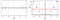# Building custom bijectors with Tensorflow Probability

## Introduction

We often need to approximate distributions using models. If the target distribution has a known form, such as a Gaussian, then we can simply find the values of the mean and variance that best fit the data. What if the data has a more complex distribution? Chances are, if you try to fit a simple distribution to complex data, the result will be mediocre. Luckily, Tensorflow Probability has straightforward tools for modelling complex distributions, via bijectors. A bijector is a Tensorflow component representing a diffeomorphism — a bijective, differentiable function — that allows us to move freely between random variables…

# Estimating feature importance, the easy way

## Introduction

Given sufficient data, machine learning models can learn complex relationships between input features and output labels. Often, we are interested in the importances of features — the relative contributions of features to predictions made by a model. Feature importances are generally not evident, but there is a straightforward way to estimate them, which I will introduce in this article.

## A simple example

In order to get an intuitive sense of how to estimate feature importances, we’ll work through an example using the Iris data set. Let’s load the data, split it, and preprocess it appropriately.

`# Imports import numpy as np import sklearn…`

# Entropy in machine learning

## A brief overview of entropy, cross-entropy, and their usefulness in machine learning

Entropy is a familiar concept in physics, where it is used to measure the amount of “disorder” in a system. In 1948, mathematician Claude Shannon expanded this concept to information theory in a paper titled, “A Mathematical Theory of Communication”. In this article, I’ll give a brief explanation of what entropy is, and why it is relevant to machine learning.

## What is entropy?

Entropy in information theory is analogous to entropy in thermodynamics. In short, entropy measures the amount of “surprise” or “uncertainty” inherent to a random variable. …

# Understanding the kernel trick

## A short explanation of the kernel trick and its relevance to SVMs

Support-vector machines (SVMs) are powerful machine learning models for regression and classification problems. One of the key concepts behind SVMs is the kernel trick, which reduces the complexity of computing nonlinear decision boundaries. Let’s figure out what this means.

Generally speaking, most data are not linearly separable. However, it is possible to transform data to higher-dimensional spaces where they are linearly separable. From there, we can easily compute linear decision boundaries. In the following picture, the green and blue points are not linearly separable. By adding a quadratic feature, however, we can separate them using the dashed red line.Transforming data to a higher-dimensional space to find a linear separation (source)

Let’s…## Romain Hardy

Machine learning engineer at Holy Grail Inc.